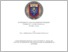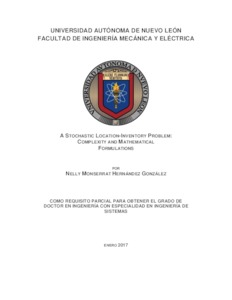# A stochastic location-inventory problem: complexity and mathematical formulations.

Hernández González, Nelly Montserrat (2017) A stochastic location-inventory problem: complexity and mathematical formulations. Doctorado thesis, Universidad Autónoma de Nuevo León.Vista previa
Texto

## Resumen

OBJECTIVE AND METHODOLOGY OF THE STUDY: The objective of this research is the study and the optimization of the Location-Inventory Problem (LIP). This problema defines how many facilities to locate, where to locate them, which retailers they serve, how manage their inventory, so as to minimize the total cost, while ensuring a specified service level. Because of the nature of the problem, an extensive variety of models can be generated. The problem addressed in this thesis consists on a mono-product supply chain of two echelons formed by a set of plants, a set of distribution centers, and a set of retailers. The most distinctive features are the stochastic demand, the consideration of multiple plants and the inventory measurement in each selected facility. The inclusion of several plants involves the presence of allocation decisions. In our case they are limited as single source, which means that each demand point should be serviced by a single supply point. This study provides the computational complexity proof of LIP, the mathematical formulation of the problem and some exact techniques for solving it. In the complexity proof, we demonstrate that the decision version of the Location-Inventory Problem is NPcomplete by building a reduction from the Bin Packing Problem, revealing that LIP is at least as difficult as the Bin Packing Problem. As consequence the Location-Inventory Problem is NP-hard. Regarding to the mathematical formulation, we present a Mixed Integer Nonlinear Problem (MINLP), which is demonstrated to be nonconvex. Therefore, two reformulations are developed, one, still, a Mixed Integer Nonlinear Problem (MINLP2) and one as a Mixed Integer Linear Problem (MILP). The nonlinear terms in MINLP2 are treated by approximations made through the secant and the pieciwese method. Concerning the MILP, it is solved by a column generation technique. Finally, we conduct an analysis for evaluating the behavior of the supply chain, and also the robustness of the solution against the uncertainty. To do that we performed a simulation that generates different sizes of demand and evaluates the feasibility of the optimal solutions to the new demand values. CONTRIBUTIONS AND CONCLUSIONS: The importance of the presented work consists on the integration of elements that, as far as we know, have not been addressed together in the literature. This integration allows to study more practical situations, but resulted in a much more complex problem, mainly due to the consideration of several plants with different parameters, the stochastic demands and the capacitated locations. Moreover, inventory management involve necessarily nonlinear terms, which make the problem even harder to solve. Some papers involve multiple plants, but they assume that the value of their parameters such as delivery time, shipping cost or production capacity are the same for all the plants. This assumption make the allocation decisions indistinct and therefore, greatly simplifies the problem In addition, none of the published works formally demonstrated the computational complexity of the general problem. It is also an important contribution of this research. The result that LIP is NP-hard suggests that any exact algorithm may fail to solve large instances, nevertheless, the strategic nature of the problem indicates that an optimal solution is valuable, since the savings between an optimal and other feasible solution can represent a large amount of money. For that reason, we decided to explore exact solution methods. The mathematical models are also a contribution. On one side, the reformulations allow to solve the problem, to optimality or near to optimality, directly with an solver without the need of decomposing the problem. On the other side, the column generation takes advantages of the structure of the problem. Computational experiments evaluate the techniques, showing that the approximation by piecisewise method generates the best results, i.e., good solutions in a reasonable time. When the instances to be solved are not too large and when the CPU time is not limited, MILP model developed in this thesis can be used .

Información adicional: Tesis (Doctor en Ingeniería con especialidad en Ingeniería de Sistemas)
Divisiones: Ingeniería Mecánica y Eléctrica
Usuario depositante: Lic. Josimar PulidoVer elemento